# Tag: Artin

The Bulletin of the AMS just made this paper by Julia Mueller available online: “On the genesis of Robert P. Langlands’ conjectures and his letter to Andre Weil” (hat tip +ChandanDalawat and +DavidRoberts on Google+).

It recounts the story of the early years of Langlands and the first years of his mathematical career (1960-1966)leading up to his letter to Andre Weil in which he outlines his conjectures, which would become known as the Langlands program.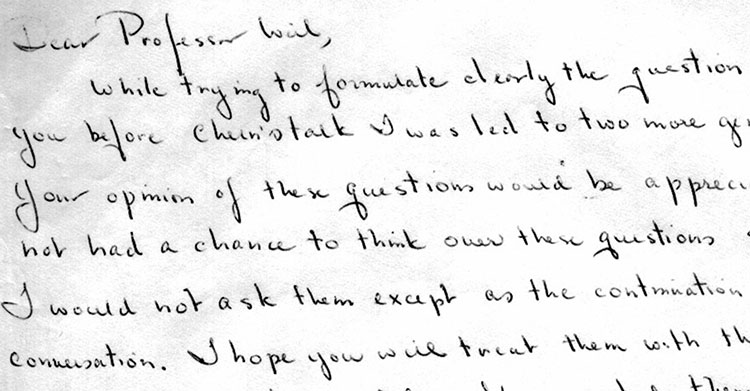Langlands letter to Weil is available from the IAS.

The Langlands program is a vast net of conjectures. For example, it conjectures that there is a correspondence between

– $n$-dimensional representations of the absolute Galois group $Gal(\overline{\mathbb{Q}}/\mathbb{Q})$, and

– specific data coming from an adelic quotient-space $GL_n(\mathbb{A}_{\mathbb{Q}})/GL_n(\mathbb{Q})$.

For $n=1$ this is essentially class field theory with the correspondence given by Artin’s reciprocity law.

Here we have on the one hand the characters of the abelianised absolute Galois group

$Gal(\overline{\mathbb{Q}}/\mathbb{Q})^{ab} \simeq Gal(\mathbb{Q}(\pmb{\mu}_{\infty})/\mathbb{Q}) \simeq \widehat{\mathbb{Z}}^{\ast}$

and on the other hand the connected components of the idele class space

$GL_1(\mathbb{A}_{\mathbb{Q}})/GL_1(\mathbb{Q}) = \mathbb{A}_{\mathbb{Q}}^{\ast} / \mathbb{Q}^{\ast} = \mathbb{R}_+^{\ast} \times \widehat{\mathbb{Z}}^{\ast}$

For $n=2$ it involves the study of Galois representations coming from elliptic curves. A gentle introduction to the general case is Mark Kisin’s paper What is … a Galois representation?.

One way to look at some of the quantum statistical systems studied via non-commutative geometry is that they try to understand the “bad” boundary of the Langlands space $GL_n(\mathbb{A}_{\mathbb{Q}})/GL_n(\mathbb{Q})$.

Here, the Bost-Connes system corresponds to the $n=1$ case, the Connes-Marcolli system to the $n=2$ case.

If $\mathbb{A}’_{\mathbb{Q}}$ is the subset of all adeles having almost all of its terms in $\widehat{\mathbb{Z}}_p^{\ast}$, then there is a well-defined map

$\pi~:~\mathbb{A}’_{\mathbb{Q}}/\mathbb{Q}^{\ast} \rightarrow \mathbb{R}_+ \qquad (x_{\infty},x_2,x_2,\dots) \mapsto | x_{\infty} | \prod_p | x_p |_p$

The inverse image of $\pi$ over $\mathbb{R}_+^{\ast}$ are exactly the idele classes $\mathbb{A}_{\mathbb{Q}}^{\ast}/\mathbb{Q}^{\ast}$, so we can view them as the nice locus of the horrible complicated quotient of adele-classes $\mathbb{A}_{\mathbb{Q}}/\mathbb{Q}^*$. And we can view the adele-classes as a ‘closure’ of the idele classes.

But, the fiber $\pi^{-1}(0)$ has horrible topological properties because $\mathbb{Q}^*$ acts ergodically on it due to the fact that $log(p)/log(q)$ is irrational for distinct primes $p$ and $q$.

This is why it is better to view the adele-classes not as an ordinary space (one with bad topological properties), but rather as a ‘non-commutative’ space because it is controlled by a non-commutative algebra, the Bost-Connes algebra.

For $n=2$ there’s a similar story with a ‘bad’ quotient $M_2(\mathbb{A}_{\mathbb{Q}})/GL_2(\mathbb{Q})$, being the closure of an ‘open’ nice piece which is the Langlands quotient space $GL_2(\mathbb{A}_{\mathbb{Q}})/GL_2(\mathbb{Q})$.

Last time we discovered that the mental picture to view prime numbers as knots in $S^3$ was first dreamed up by David Mumford. Today, we’ll focus on where and when this happened.

3. When did Mazur write his unpublished preprint?

According to his own website, Barry Mazur did write the paper Remarks on the Alexander polynomial in 1963 or 1964. A quick look at the references gives us a coarse lower- and upper-estimate.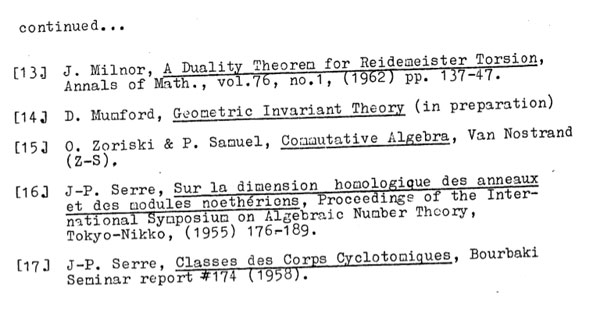Apart from a paper by Iwasawa and one by Milnor, all references predate 1962 giving us a lower-bound. More interesting is reference (14) to David Mumford’s Geometric Invariant Theory (GIT) which was first published in 1965 and is referred to as ‘in preparation’, so the paper was written no later than 1965. If we look a bit closer we see than some GIT-references are very preciseindicating that Mazur must have had the final version of GIT to consult, making it rather difficult to believe that the preprint was written late 1963 or early 1964.

Mazur’s dating of the preprint is probably based on this penciled note on the frontpage of the only surviving copy of the preprint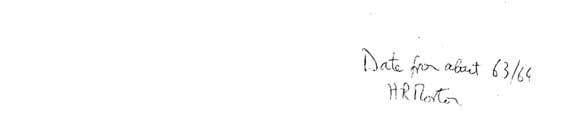It reads : “Date from about 63/64, H.R. Morton”. Hugh Morton of Liverpool University confirms that it is indeed his writing on the preprint.

Further, he told me that early 64 Christopher Zeeman held a Topology Symposium in Cambridge UK, where Hugh was a graduate student at the time and, as far as he could recall, Mazur attended that conference and gave him the preprint on that occasion, whence the 63/64 dating. Hugh kindly offered to double-check this with Terry Wall who cannot remember Mazur attending that particular conference.

In fact, we will see that a more correct dating of the Mazur-preprint will be : late 1964 or early 1965.

4. The birthday : July 10th 1964

Clearly, Mumford’s insight predates the Mazur-preprint. In the first section, Mazur mentions ‘Grothendieck cohomology groups’ rather than ‘Etale cohomology groups’.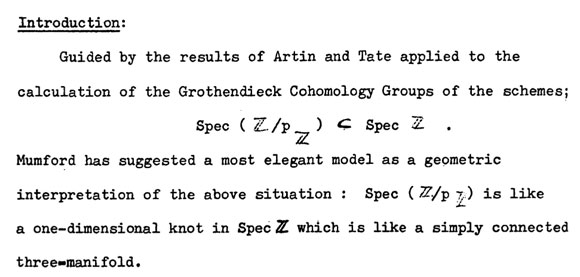At the time, Artin’s seminar notes on Grothendieck topologies (spring 1962) were widely distributed, and Artin and Grothendieck were in the process of developing etale cohomology in their Paris 1963/64 seminar SGA 4, while Mumford was working on GIT in Harvard.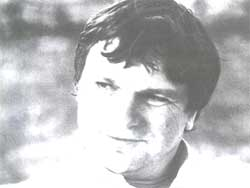Mike Artin, David Mumford and Jean-Louis Verdier all attended the Woods Hole conference from july 6 till july 31 1964, famous for producing the Atiyah-Bott fixed point theorem (according to Fulton first proved by Verdier at the conference).

Etale cohomology was a hot topic at that conference. On july 10th there were three talks, Artin spoke on ‘Etale cohomology of schemes’, Verdier on ‘A duality theorem in the etale cohomology of schemes’ and John Tate on ‘Etale cohomology over number fields’.

After a first week of talks, more informal seminars were organized, including the Atiyah-Bott seminar leading to the ‘Woods hole duality theorem’ and one by Lubin-Tate and Serre on elliptic curves and formal groups. Two seminars adressed Etale Cohomology.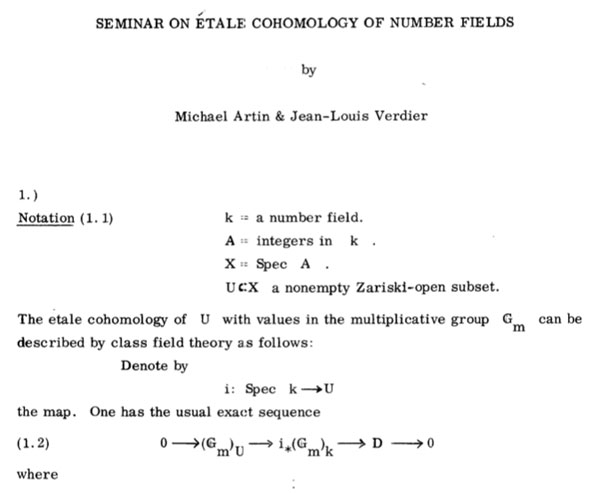Artin and Verdier ran a seminar on the etale cohomology of number fields leading to their duality result, and, three young turks : Daniel Quillen, Steve Kleiman and Robin Hartshorne ran a Baby Seminar on Etale cohomology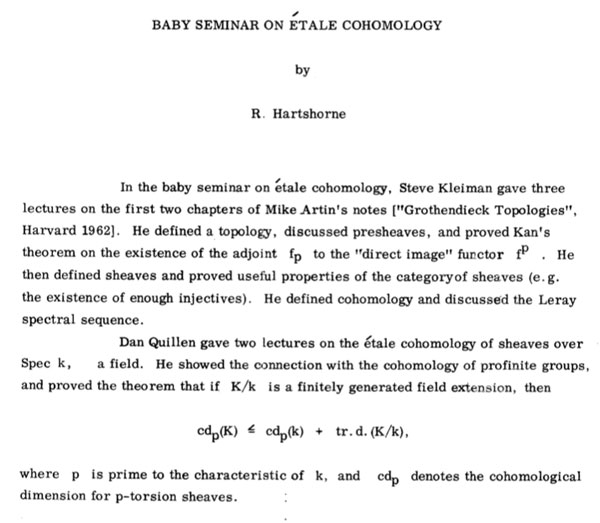Probably it is safe to say that the talks by Artin, Verdier and Tate on July 10th sparked the primes=knots idea, and if not then, a couple of days later.

5. The birthplace : the Whitney Estate

The ‘Woods Hole’ conference took place at the Whitney Estate and all the lectures took place in the rustic rooms of the main building and the participants (and their families) were housed in rented cottages in the neighborhood, for the duration of the summer.

The only picture i managed to find from the Whitney house comes from a rather surprising source : Gardeners and Caretakers ofWoods Hole. Anyway, here it is :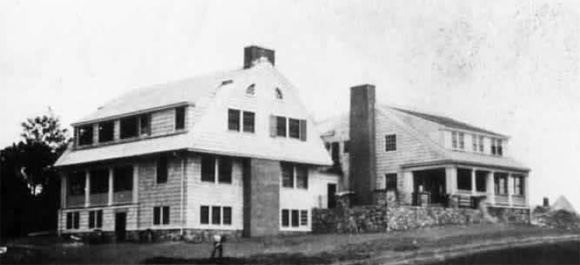Probably, the knots=primes analogy was first dreamed up inside, or in the immediate neighborhood, on a walk to or from the cottages, overlooking the harbor.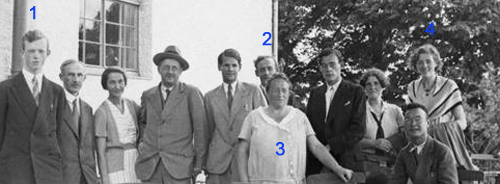For the better part of the 30ties, Ernst Witt (1) did hang out with the rest of the ‘Noetherknaben’, the group of young mathematicians around Emmy Noether (3) in Gottingen.

In 1934 Witt became Helmut Hasse‘s assistent in Gottingen, where he qualified as a university lecturer in 1936. By 1938 he has made enough of a name for himself to be offered a lecturer position in Hamburg and soon became an associate professor, the down-graded position held by Emil Artin (2) until he was forced to emigrate in 1937.

A former fellow student of him in Gottingen, Erna Bannow (4), had gone earlier to Hamburg to work with Artin. She continued her studies with Witt and finished her Ph.D. in 1939. In 1940 Erna Bannow and Witt married.

So, life was smiling on Ernst Witt that sunday january 28th 1940, both professionally and personally. There was just one cloud on the horizon, and a rather menacing one. He was called up by the Wehrmacht and knew he had to enter service in february. For all he knew, he was spending the last week-end with his future wife… (later in february 1940, Blaschke helped him to defer his military service by one year).

Still, he desperately wanted to finish his paper before entering the army, so he spend most of that week-end going through the final version and submitted it on monday, as the published paper shows.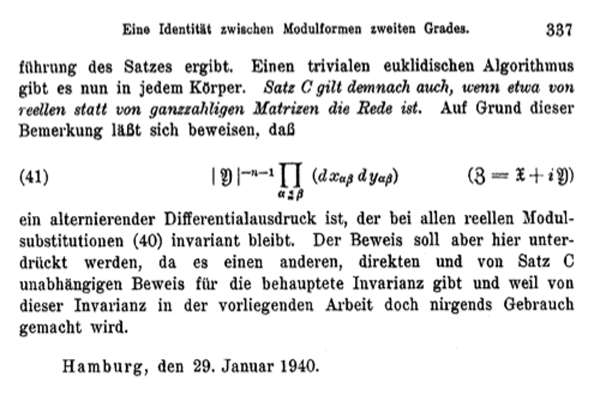In the 70ties, Witt suddenly claimed he did discover the Leech lattice ${\Lambda}$ that sunday. Last time we have seen that the only written evidence for Witt’s claim is one sentence in his 1941-paper Eine Identität zwischen Modulformen zweiten Grades. “Bei dem Versuch, eine Form aus einer solchen Klassen wirklich anzugeben, fand ich mehr als 10 verschiedene Klassen in ${\Gamma_{24}}$.”

But then, why didn’t Witt include more details of this sensational lattice in his paper?

Ina Kersten recalls on page 328 of Witt’s collected papers : “In his colloquium talk “Gitter und Mathieu-Gruppen” in Hamburg on January 27, 1970, Witt said that in 1938, he had found nine lattices in ${\Gamma_{24}}$ and that later on January 28, 1940, while studying the Steiner system ${S(5,8,24)}$, he had found two additional lattices ${M}$ and ${\Lambda}$ in ${\Gamma_{24}}$. He continued saying that he had then given up the tedious investigation of ${\Gamma_{24}}$ because of the surprisingly low contribution

$\displaystyle | Aut(\Lambda) |^{-1} < 10^{-18}$

to the Minkowski density and that he had consented himself with a short note on page 324 in his 1941 paper.”

In the last sentence he refers to the fact that the sum of the inverse orders of the automorphism groups of all even unimodular lattices of a given dimension is a fixed rational number, the Minkowski-Siegel mass constant. In dimension 24 this constant is

$\displaystyle \sum_{L} \frac{1}{| Aut(L) |} = \frac {1027637932586061520960267}{129477933340026851560636148613120000000} \approx 7.937 \times 10^{-15}$

That is, Witt was disappointed by the low contribution of the Leech lattice to the total constant and concluded that there might be thousands of new even 24-dimensional unimodular lattices out there, and dropped the problem.

If true, the story gets even better : not only claims Witt to have found the lattices ${A_1^{24}=M}$ and ${\Lambda}$, but also enough information on the Leech lattice in order to compute the order of its automorphism group ${Aut(\Lambda)}$, aka the Conway group ${Co_0 = .0}$ the dotto-group!

Is this possible? Well fortunately, the difficulties one encounters when trying to compute the order of the automorphism group of the Leech lattice from scratch, is one of the better documented mathematical stories around.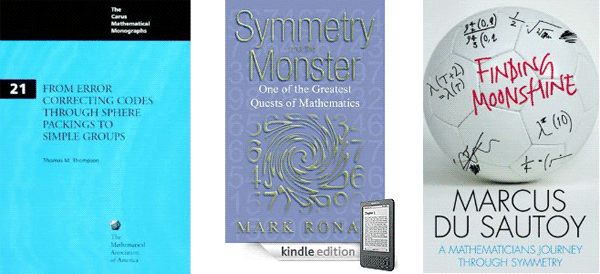The books From Error-Correcting Codes through Sphere Packings to Simple Groups by Thomas Thompson, Symmetry and the monster by Mark Ronan, and Finding moonshine by Marcus du Sautoy tell the story in minute detail.

It took John Conway 12 hours on a 1968 saturday in Cambridge to compute the order of the dotto group, using the knowledge of Leech and McKay on the properties of the Leech lattice and with considerable help offered by John Thompson via telephone.

But then, John Conway is one of the fastest mathematicians the world has known. The prologue of his book On numbers and games begins with : “Just over a quarter of a century ago, for seven consecutive days I sat down and typed from 8:30 am until midnight, with just an hour for lunch, and ever since have described this book as “having been written in a week”.”

Conway may have written a book in one week, Ernst Witt did complete his entire Ph.D. in just one week! In a letter of August 1933, his sister told her parents : “He did not have a thesis topic until July 1, and the thesis was to be submitted by July 7. He did not want to have a topic assigned to him, and when he finally had the idea, he started working day and night, and eventually managed to finish in time.”

So, if someone might have beaten John Conway in fast-computing the dottos order, it may very well have been Witt. Sadly enough, there is a lot of circumstantial evidence to make Witt’s claim highly unlikely.

For starters, psychology. Would you spend your last week-end together with your wife to be before going to war performing an horrendous calculation?

Secondly, mathematical breakthroughs often arise from newly found insight. At that time, Witt was also working on his paper on root lattices “Spiegelungsgrupen and Aufzähling halbeinfacher Liescher Ringe” which he eventually submitted in january 1941. Contained in that paper is what we know as Witt’s lemma which tells us that for any integral lattice the sublattice generated by vectors of norms 1 and 2 is a direct sum of root lattices.

This leads to the trick of trying to construct unimodular lattices by starting with a direct sum of root lattices and ‘adding glue’. Although this gluing-method was introduced by Kneser as late as 1967, Witt must have been aware of it as his 16-dimensional lattice ${D_{16}^+}$ is constructed this way.

If Witt wanted to construct new 24-dimensional even unimodular lattices in 1940, it would be natural for him to start off with direct sums of root lattices and trying to add vectors to them until he got what he was after. Now, all of the Niemeier-lattices are constructed this way, except for the Leech lattice!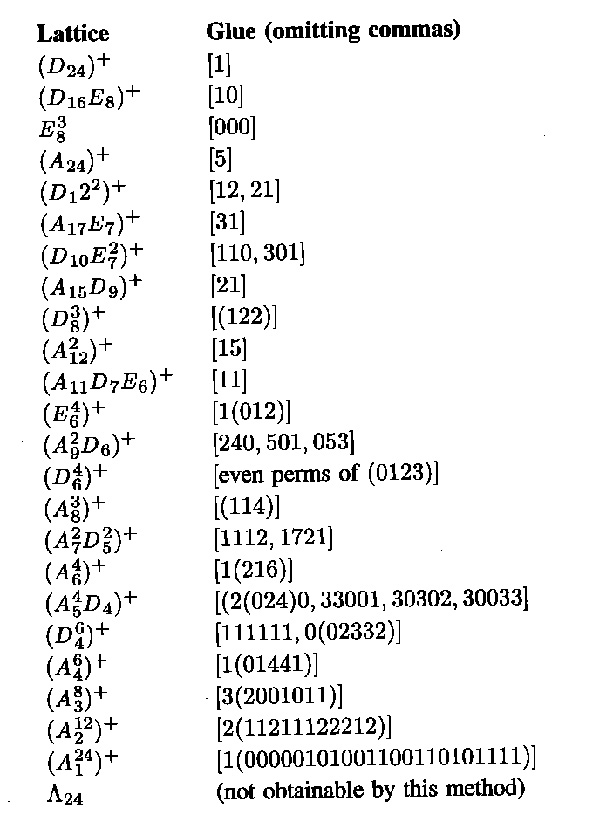I’m far from an expert on the Niemeier lattices but I would say that Witt definitely knew of the existence of ${D_{24}^+}$, ${E_8^3}$ and ${A_{24}^+}$ and that it is quite likely he also constructed ${(D_{16}E_8)^+, (D_{12}^2)^+, (A_{12}^2)^+, (D_8^3)^+}$ and possibly ${(A_{17}E_7)^+}$ and ${(A_{15}D_9)^+}$. I’d rate it far more likely Witt constructed another two such lattices on sunday january 28th 1940, rather than discovering the Leech lattice.

Finally, wouldn’t it be natural for him to include a remark, in his 1941 paper on root lattices, that not every even unimodular lattices can be obtained from sums of root lattices by adding glue, the Leech lattice being the minimal counter-example?

If it is true he was playing around with the Steiner systems that sunday, it would still be a pretty good story he discovered the lattices ${(A_2^{12})^+}$ and ${(A_1^{24})^+}$, for this would mean he discovered the Golay codes in the process!

Which brings us to our next question : who discovered the Golay code?